# Walking

Lucie can walk about 3 4/5 miles each hour. How far can she walk in 2 hours 45 minutes?

x =  10.45 mi

### Step-by-step explanation: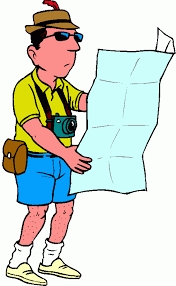Did you find an error or inaccuracy? Feel free to write us. Thank you!Tips to related online calculators
Need help to calculate sum, simplify or multiply fractions? Try our fraction calculator.
Do you want to convert length units?
Do you want to convert velocity (speed) units?
Do you want to convert time units like minutes to seconds?

## Related math problems and questions:

• Constant motionThe car has undergone constant motion 120 km in 1 hour 30 minutes. Determine its average speed. Determine how long the car drove in the first 20 minutes and 50 minutes from the start of the move.
• Cars 6At 9:00 am two cars started from the same town and traveled at a rate of 35 miles per hour and the other car traveled at a rate of 40 miles per hour. After how many hours will the cars be 30 miles apart?
• Cyclist and carA cyclist who runs at a speed of 20km per hour will arrive at the finish in 4.5 hours. How fast does the car have to drive the same route in 2 hours?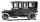Car started at 11:40 at speed 54 km/h from A and to B arrived at 12:20. Determine the distance A and B.
• Walking woman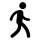Reeta walks 5/7 km in 1 hour. How far does she go in 3 1/2 hours?
• Two vans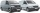At 8:30 a. M. , two vans leave at a parking area. They travel in opposite directions: one van travels at 48 km/h and the other at 62 km/h. At what time will the cars be 550 km apart?
• RunningJoanne ran 8 1/2 Kyleigh 1 2/3 and John 6 3/4 miles in one day. How many miles did the girls run in 3 days?
• Train and walker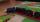Walker passes an hour 5 km. Express train per hour 100 km. Walker went 4 hours. How many minutes took the distance express train?
• Truck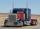Truck went for 4 hours and 30 minutes. If truck increase speed by 11 km/h, went same distance in 2 hours. Calculate this distance.
• Two trains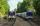Two trains go at each other. First at speed 40 km/h and the second at speed 60 km/h. At what distance will be 30 minutes before they will meet?
• The caravans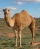At 6:00 in the morning, a caravan set out of the oasis at a speed of 1.5 miles per hour. Two hours later, a second caravan followed her at 2.5 miles per hour. When did the faster caravan run slower? How far from the oasis?
• Cyclist 9A cyclist travels at a speed of 4.25 km per hour. At that rate, how far can he travel in 3.75 hours?
• Motion against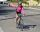From two places 36 km apart, a pedestrian and a cyclist will go straight opposite each other. How long will they meet if the speed of the pedestrian is 4 km/h and the cyclist 12 km/h?
• Time clockWhat are the angle of the hour hand and the minute hand if it is 0:40?
• Cruise liner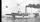Cruise liner runs along the river between points A and B. The journey downstream takes 40 minutes 1 hour upriver. The flow rate of the river is 3 km/h. What is the speed of cruise liner?
• The touristThe tourist came started from the hostel at an average speed of 5km/h. Half an hour later, the bicyclist started along the same route at a speed of 20km/h. How many minutes will a cyclist catch up and how many kilometers will he go?
• Two friendsTwo friends on a bicycle rode against each other from two places 49 km apart. The first set off at 8.00 at a speed of 20km/h, the second 12 minutes later at a speed of 25km/h. What time do they meet? How many km will each of them travel since then?